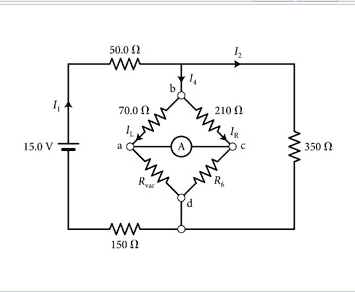ForumPages: 1Working with Current in Wheatstone Bridge (Read 2815 times)
 evan123 New MemberOfflinePosts: 5Working with Current in Wheatstone Bridge Feb 18th, 2018, 12:26am   1. The problem statement, all variables and given/known data The ammeter in the Wheatstone bridge of measures zero current when the resistance Rvar of the variable resistor is set to 193Ω . What is the current IL through the left side of the bridge? 2. Relevant equations V1 + V2 + V3 + V4 + ..... + VN = E Rx = Rvar*R3/R13. The attempt at a solution To start, I calculated the equivalent resistance of the diamond part in the center, which is ( 1 70 + 139 + 1 210 + 579 ) − 1 (170+139+1210+579)−1, where I calculated the R6 to be 579 Ω. I used this and V1 + V2 + V3 + V4 + ..... + VN = E to find the voltage across the whole diamond part (using Kirchoff's Current Rule). The current into the diamond should be equivalent to the one out of it, 0.04 A. Using this knowledge, and the voltage I calculated across the diamond part, and divided it by the equivalent resistance on the left side, which should give me current. I found the current on the right side the same way, and sure enough they added to 0.04 A. I got an answer of 0.02382 A for the left side, which is wrong. Back to topIP LoggedPages: 1